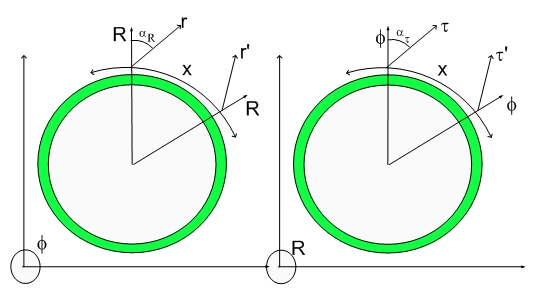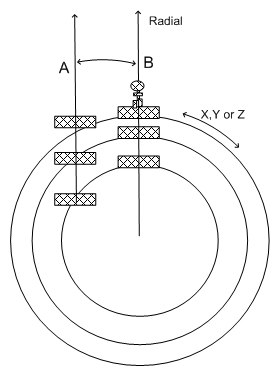Viewing posts from July, 2007

## Comments #1 on The Silver SurferComments on The Silver Surfer and The Hypergeometrical Universe

Anonymous said...

Say, there are two observers A and B separated by distance X. The observer A starts motion in direction of B with such an impulse, that by the time he reaches B, his velocity will match the Hubble velocity. What will the observer B see? Will the A continue his motion? Will he slow down and stop?
---------------------------------------------------
---------------------------------------------------
---------------------------------------------------
To answer this question the first thing to notice is that one can see A and B on both cross-sections shown above. We will use just the left panel RXYZ cross-section to answer this question.
Let's first check the initial condition where the Fabric of Space is relaxed on both A and B positions, reminding that the Fabric of Space is the wave we ride - where the 3D space and all dilators (matter) are located.

At first sight you might think that the objects on positions A and B are at rest with respect to one another. This would be the case if the 3D Hypersurface were not expanding at the speed of light. In reality they are traveling away from each other at their Hubble velocity. Be their distance X, then they are running away from each other by X/R*cThis can be easily derived if one considers that the radial expansion takes place at the speed of light and the geometry of the figure.

That is why one can only see thing up to one radian in each direction.
The first thing to notice is that it is not clear how to measure the local deformation of the Fabric of Space. This topology (hyperspherical expanding 3D Universe) is connected to the 4D Big Bang proposed in the Cosmogenesis paper.
Under that assumption (Big Bang = 4D explosion), the average velocity on any region of the 3D space is the Hubble velocity, that is, farther regions contains celestial objects that on average run away from us at the Hubble speed. What one can distinguish from one region to another is the width of that distribution. When most of the interactions had taken place a long time ago, the distribution will be sharper. A sharper distribution of receding velocites means that the objects are close to their Fabric of Space relaxed positions.
The comment requires that object A, under the action of an impulse, to reach its Hubble velocity at position B. Velocity is a tilt in the local Fabric of Space and that tilt should make the local normal to be parallel to the Fabric of Space normal at position B. The diagram that would reflect that condition would be the following:For the distance between the objects to diminish, the tilt (velocity) has to be larger than the Hubble tilt (velocity) between the two objects, thus the proposed experiment doesn't make sense and that is the reason for the paradox.

## Encounters of the 4th Kind....:)